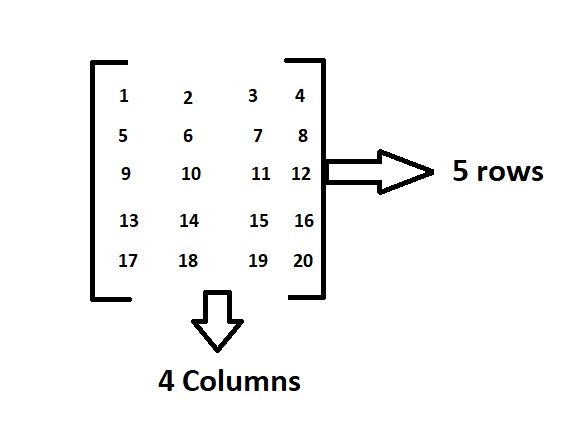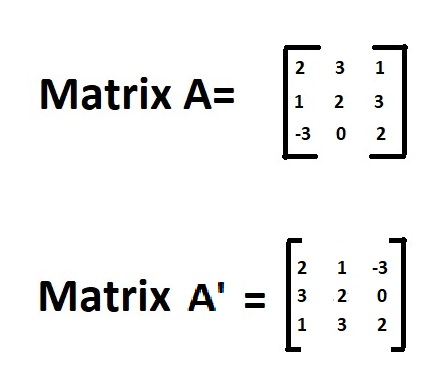# Program for Transpose a Matrix in Python & C++ Programming

In the previous article, we have discussed about Program for addition of two matrices in Python & C++ Programming. Let us learn Program for Transpose a Matrix in C++ Program and Python.

What is a matrix:

A matrix is a rectangular sequence of numbers divided into columns and rows. A matrix element or entry is a number that appears in a matrix.

Example:Above is the matrix which contains 5 rows and 4 columns and having elements from 1 to 20.

In this order, the dimensions of a matrix indicate the number of rows and columns.

Here as there are 5 rows and 4 columns it is called as 5*4 matrix.

What is Matrix Transpose:

The interchanging of rows and columns is known as a matrix transpose. It’s abbreviated as A’. The element in A’s ith row and jth column will be moved to A’s jth row and ith column.Examples for matrix Transpose:

Input:

Matrix 1 = 2 3 1
1 2 3

Output:

Printing the transpose of matrices :
2 1
3 2
1 3

## Program for Matrix Transpose

Explore more instances related to python concepts from Python Programming Examples Guide and get promoted from beginner to professional programmer level in Python Programming Language.

### Method #1:Using nested Loops in Python

A matrix can be implemented as a nested list in Python (list inside a list). Each variable can be thought of as a row in the matrix.

Approach:

• Create two two-dimensional arrays A, and set their values. (In this case, we used a  initialized statically)
• Calculate the number of rows and columns in the array A and store it in the variables rows and columns.
• Declare a new array transpose, this time with dimensions in columns an rows(in reverse order).
• Calculate the transpose matrix by initializing transpose[i][j]=A[j][i].
• Print the transpose matrix.

Below is the implementation:

# given matrix A
A = [[2, 3, 1],
[1, 2, 3]]
rows = len(A)
columns = len(A)
# Initialize the transpose of matrices elements to 0
# with rows as columns and columns as rows as dimensions
matrixTrans = [[0, 0],
[0, 0],
[0, 0]]
# iterate through rows
for i in range(rows):
# iterate through columns
for j in range(columns):
matrixTrans[j][i] = A[i][j]
# printing the transpose of matrices
print("Printing the transpose of matrices : ")
for rows in matrixTrans:
print(*rows)


Output:

Printing the transpose of matrices :
2 1
3 2
1 3

### Method #2:Using List Comprehension in Python

The program’s output is the same as previously. For iterating through each element in the array, we used the nested list comprehension.

Understanding the list helps us to write concise codes and we need to always try to use them in Python. They’re really beneficial.

Below is the implementation:

# given matrix A
A = [[2, 3, 1],
[1, 2, 3]]
rows = len(A)
columns = len(A)
# Initialize the transpose of matrices elements to 0
# with rows as columns and columns as rows as dimensions
matrixTrans = [[0, 0],
[0, 0],
[0, 0]]
# using list comprehension to transpose a matrix
matrixTrans = [[A[j][i] for j in range(rows)] for i in range(columns)]
# printing the transpose of matrices
print("Printing the transpose of matrices : ")
for rows in matrixTrans:
print(*rows)


Output:

Printing the transpose of matrices :
2 1
3 2
1 3

### Method #3:Using nested loops in C++

We used nesting loops in this program to iterate through and row and column.

Calculate the transpose matrix by initializing transpose[i][j]=A[j][i].In the  the appropriate elements and store them in the result at each level.

Let us take dynamic input in this case.

Below is the implementation:

#include <iostream>
using namespace std;
int main()
{
int rows, columns, A, matrixTrans, i, j;
cout << "Enter the number of rows of the matrix "<<endl;
cin >> rows;
cout << "Enter the number of columns of the matrix"<<endl;
cin >> columns;
cout << "Enter the elements of the matrix " << endl;
// Initializing matrix A with the user defined values
for(i = 0; i < rows; ++i)
for(j = 0; j < columns; ++j)
{
cout << "Enter element A" << i + 1 << j + 1 << " = ";
cin >> A[i][j];
}

// Calculating transpose of the matrix
for(i = 0; i < rows; ++i)
for(j = 0; j < columns; ++j)
matrixTrans[j][i] = A[i][j];
//printing matrix A
cout << endl << " printing the matrix A" << endl;
for (i = 0; i < rows; ++i) {
for (j = 0; j < columns; ++j) {
cout << A[i][j] << "  ";
}
cout << endl;
}

//printing the transpose of matrices
cout << endl << " printing the transpose of matrices A " << endl;

for (i = 0; i < columns; ++i) {
for (j = 0; j < rows; ++j) {
cout << matrixTrans[i][j] << "  ";
}
cout << endl;
}
return 0;
}

Output:

Enter the number of rows of the matrix
5
Enter the number of columns of the matrix
4
Enter the elements of the matrix
Enter element A11 = 1
Enter element A12 = 2
Enter element A13 = 3
Enter element A14 = 4
Enter element A21 = 5
Enter element A22 = 8
Enter element A23 = 9
Enter element A24 = 7
Enter element A31 = 2
Enter element A32 = 1
Enter element A33 = 6
Enter element A34 = 4
Enter element A41 = 5
Enter element A42 = 2
Enter element A43 = 8
Enter element A44 = 0
Enter element A51 = -5
Enter element A52 = 3
Enter element A53 = 4
Enter element A54 = 8

printing the matrix A
1 2 3 4
5 8 9 7
2 1 6 4
5 2 8 0
-5 3 4 8

printing the transpose of matrices A
1 5 2 5 -5
2 8 1 2 3
3 9 6 8 4
4 7 4 0 8

Related Programs: